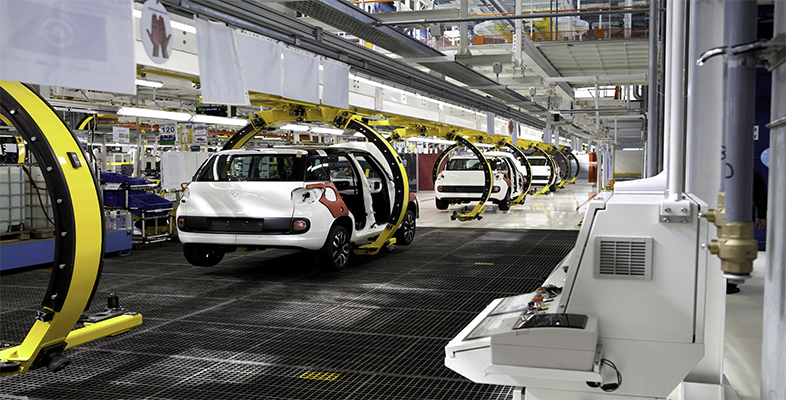### Become an OU studentAssessing risk in engineering, work and life

Start this free course now. Just create an account and sign in. Enrol and complete the course for a free statement of participation or digital badge if available.

# Probability of failure

The probability of a component failing is calculated in a similar way. However, it can be hard to assess what this probability means, in practical terms. For instance, suppose that an oil storage tank is fitted with a level-detecting device that shuts off the valve that lets oil into the tank when it is full. An assessment of the device suggests that there is a 1 in 10 000 probability that it will fail each time the tank is filled. If the tank is filled every day, how often is failure likely?

This isn’t a question you can answer, since the device might never fail. What you can say is that after a given interval, there is a particular probability of failure. For instance, you could assess the probability of a failure within 25 years. It will be assumed here that regular maintenance checks ensure that the device does not deteriorate significantly, so that the probability of failure stays constant over time.

To calculate this, consider what the probability would be if the tank were filled twice. That would give three possible outcomes: the level detector doesn’t fail, it fails once or it fails twice. Since for any event , , the calculation that the level detector doesn’t fail on any single occasion is 1 minus the probability that it does fail – that is,

Now you have the probability of no failure for one occasion. How about two occasions? That is given by the product of the two individual probabilities, which is 0.99992 in this case. Working this way, you avoid any complications from multiple failures entering the calculation.

So how about the probability over 25 years? In 25 years, the number of times the tank will be filled is the number of days, 25 × 365 = 9125 (ignoring leap years). It is acceptable to round this to 9000 without having to worry about accuracy, but you cannot round the 0.9999 or your answer will always be 1. So you need to calculate 0.99999000 to estimate the probability of no failures:

This is the probability of no failures, so the probability that there is a failure is given by 1 − 0.41 = 0.59. So there is a 59% probability of failure during 25 years of operation.

If the Health and Safety regulators decided that failure of the device could trigger an event similar to that at Buncefield, they might require the operators to fit a second device (the same as the first) to the tank. For the tank to overfill now requires both devices to fail at the same time.

## Activity 7 Probability of failure

Timing: Allow approximately 15 minutes.

With two safety devices installed, calculate the probability that both devices fail at the same time within 25 years.